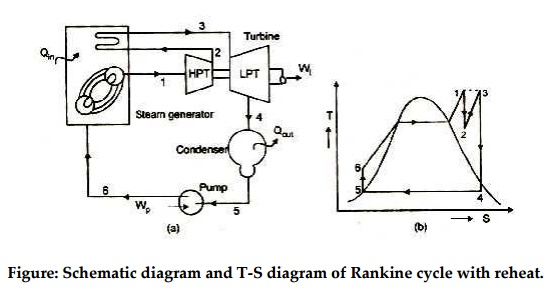Home | | Power Plant Engineering | Process of the Rankine Cycle

# Process of the Rankine Cycle

Type of Basic Boilers thermodynamic cycles process of the Rankine cycle, Real Rankine Cycle variation of Basic Rankine Cycle

Type of Basic Boilers thermodynamic cycles  process of the Rankine cycle

BOILER CYCLES

In general, two important area of application for thermodynamics are:

1. Power generation

2. Refregeration

Both are accomplished by systems that operate in thermodynamic cycles such as:

a.     Power cycles: Systems used to produce net power output and are often called engines.

Refrigeration cycles: Systems used to produce refregeration effects are called refregerators2. Vapour power cycles

In this case, the working fluid exists in the vapour phase during one part of the cycle and in the liquid phase during another part.

Vapour power cycles can be categorized as

a.     Carnot cycle

b.    Rankine cycle

c.      Reheat cycle

d.    Regenerative cycle

e.     Binary vapour cycle

Steam cycles (Ranking cycle)

The Rankine cycle is a thermodynamic cycle. Like other thermodynamic cycle, the maximum efficiency of the Ranking cycle is given by calculating the maximum efficiency of the carnot cycle.

Process of the Rankine CycleFigure: Schematic rep

There are four processes in the Rankine cycle, each changing the state of the working fluid. These states are identified by number in the diagram above.

Process 3-4:

First, the working fluid (water) is enter the pump at state 3 at saturated liquid and it is pumped (ideally isentropically) from low pressure to high (operating) pressure of boiler by a pump to the state 4. During this isentropic compression water temperature is slightly increased. Pumping requires a power input (for example, mechanical or electrical). The conservation of energy relation for pump is given as

Wpump = m (h4 - h3)

Process 4-1:

The high pressure compressed liquid enters a boiler at state 4 where it is heated at constant pressure by an external heat source to become a saturated vapour at statel’which in turn superheated to state 1 through super heater. Common heat source for power plant systems are coal (or other chemical energy), natural gas, or nuclear power. The conservation of energy relation for boiler is given as

Qin =m (h1 - h4)

Process 1 –2:

The superheated vapour enter the turbine at state 1 and expands through a turbine to generate power output. Ideally, this expansion is isentropic. This decreases the temperature and

pressure of the vapour at state 2. The conservation of energy relation for turbine is given as

Wturbine = m (h1 –h2)

Process 2 –3:

The vapour then enters a condenser at state 2. At this state, steam is a saturated liquid-vapour mixture where it is cooled to become a saturated liquid at state 3. This liquid then re-enters the pump and the cycle is repeated. The conservation of energy relation for condenser is given as

Qout = m (h2 –h3)

The exposed Rankine cycle can also prevent vapour overheating, which reduces the amount of liquid condensed after the expansion in the turbine.

Description

Rankine cycles describe the operation of steam heat engines commonly found in power generation plants. In such vapour power plants, power is generated by alternatively vaporizing ng fluid (in many cases water, although refrigerants such as ammonia in a Rankine cycle follows a closed loop and is re-used constantly. Water vapour seen billowing from power plants is evaporating cooling water, not working fluid. (NB: steam is invisible until it comes in contact with cool, saturated air, at which point it condenses and forms the white billowy clouds seen leaving cooling towers).Variables

Qin- heat input rate (energy per unit time) m= mass flow rate (mass per unit time)

W- Mechanical power used by or provided to the system (energy per unit time)

h- thermodynamic efficiency of the process (power used for turbine per heat input, unit

less).

The thermodynamic efficiency of the cycle as the ratio of net power output to heat input.

Wnet  =(Wturbine  -Wpump )or (Qin -Qout )

h=Wnet / Qin

Real Rankine Cycle variation of Basic Rankine Cycle

Real Ranking Cycle (Non-ideal)Figure:

In a real Rankine cycle, the compression by the pump and the expansion in the turbine are not isentropic. In other words, these processes are non-reversible and entropy is increased during the two process (indicated in the figure). This somewhat increases the power required by the pump and decreases the power generated by the turbine. It also makes calculations more involved and difficult.

Variation of the Basic Rankine Cycle:

Two main variations of the basic Rankine cycle to improve the efficiency of the steam cycles are done by incorporating Reheater and Regenerator in the ideal ranking cycle.

Rankine cycle with reheatFigure: Schematic diagram and T-S diagram of Rankine cycle with reheat.

In this variation, two turbines work in series. The first accepts vapour from the boiler at high pressure. After the vapour has passed through the first turbine, it re-enters the boiler and is reheated before passing through a second, lower pressure turbine. Among other advantages, this prevents the vapour from condensing during its expansion which can seriously damage the turbine blades.

Study Material, Lecturing Notes, Assignment, Reference, Wiki description explanation, brief detail
Mechanical and Electrical : Power Plant Engineering : Coal Based Thermal Power Plants : Process of the Rankine Cycle |